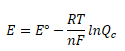### Physical Chemistry - Brief look at the Nernst equation

In the chapter of electrochemistry, the Nernst equation is in the grey area of the syllabus.
So let us look briefly at what is the Nernst equation.

## Understanding the Nernst Equation

The Nernst equation finds the cell potential of a reaction, or how much "power" a reaction will have at any given moment. You can use the equation to find out how much juice is in your battery.
Below is the general formula for the Nernst equation. You'll need to refer to it again when we perform our sample calculations.You may be wondering what all those letters in the equation mean. To decipher them, take a look at the key below:
• E: refers to the cell potential, or the amount of voltage, the reaction will have under set conditions.
• Eo: refers to the cell potential, or voltage, the reaction will have under set-standard conditions.
• R: refers to the ideal gas constant, which is 8.314 J/mol-K.
• T: refers to the temperature in Kelvins (K); room temperature equals 298 K.
• n: refers to the number of moles of transferrable electrons used to balance the equation.
• F: refers to Faraday's constant, or the charge on a mole of electrons, which is 95484.56 C/mol.
• ln: is a mathematical action called the natural log.
• Qc: refers to the reaction quotient at a given moment - found in the chapter of chemical equilibrium.
You can calculate the reaction quotient by looking at the concentration of the products and the concentrations of the reactants. To get Qc, you divide the concentration of the products by the concentration of the reactants.

-- Information found from internet.